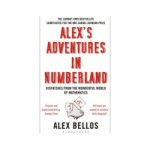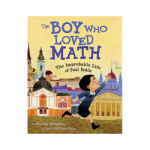# Mathematics

## Curriculum Intent

The Mathematics curriculum at the Maritime Academy has been designed to continue to build upon previous learning from Key Stage 2 and support the transition into Key Stage 3, ensuring all areas of the national curriculum are covered. Mathematics is taught using a mastery approach, whereby we teach mathematics for understanding. The curriculum is designed to ensure pupils understand basic concepts, methods and strategies and use these as building blocks to further extend learning.

Lessons are designed to enable learners to develop mathematical fluency and the ability to reason mathematically. Pupils regularly use mathematical language to strengthen conceptual understanding by enabling students to explain, reason and prove.### KS3 Maths Curriculum Overview

Images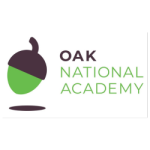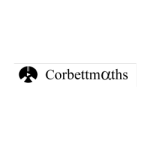Corbett MathsMaths Genie

https://www.mathsgenie.co.uk/gcse.htmlMr Barton Maths

Mr Barton Maths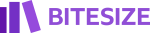Maths BBC Bitesize

KS3 Maths - BBC Bitesize

Images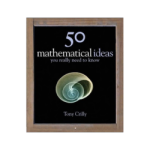50 Mathematical Ideas You Really Need to Know

by Tony Crilly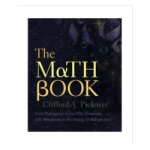The Math Book

by Clifford A Pickover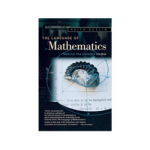The Language of Mathematics

by Keith Devlin